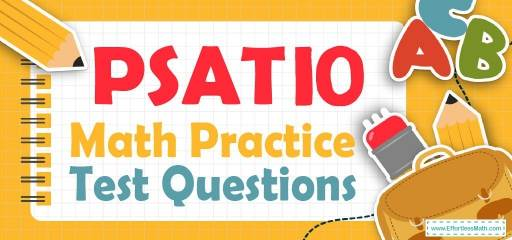# PSAT 10 Math Practice Test QuestionsAre you preparing for your PSAT 10 Math test? Review these free PSAT 10 Math Practice questions to help you learn more about PSAT 10 Math test. Reviewing practice questions is the best way to brush up on your Math skills. Here, we walk you through solving 10 common PSAT 10 Math practice problems covering the most important math concepts on the PSAT 10 Math test.

These PSAT 10 Math practice questions are designed to be similar to those found on the real PSAT 10 Math test. They will assess your level of preparation and will give you a better idea of what to study for your exam.

## 10 Sample PSAT 10 Math Practice Questions

1- The area of a circle is $$64π$$. What is the circumference of the circle?

A. $$8π$$

B. $$16π$$

C. $$32π$$

D. $$64π$$

2- Which of the following values for $$x$$ and $$y$$ satisfy the following system of equations?
$$x+4y=10$$
$$5x+10y=20$$

A. $$x=3,y=2$$

B. $$x=2,y=-3$$

C. $$x=-2,y=3$$

D. $$x=3,y=-2$$

3- If $$a ≠ 0$$ and $$6b=5a\sqrt{3}$$, then what is the value of $$\frac{2b \sqrt{3}}{4a}$$ ?

A. $$\frac{5}{4}$$

B. $$\frac{5}{4}a$$

C. $$2a$$

D. $$2a\sqrt{3}$$

4- If a gas tank can hold 25 gallons, how many gallons does it contain when it is $$\frac{2}{5}$$ full?

A. 50

B. 125

C. 62.5

D. 10

5- In the $$xy$$-plane, the point $$(4,3)$$ and $$(3,2)$$ are on line $$A$$. Which of the following equations of lines is parallel to line $$A$$?

A. $$y = 3x$$

B. $$y = \frac{x}{2}$$

C. $$y = 2x$$

D. $$y = x$$

6- In the following graph, which of the data point is farthest from the line of best fit (not shown)?

A. (6,1)

B. (5,4)

C. (3,3)

D. (2,2)

7- A football team won exactly $$80\%$$ of the games it played during the last session. Which of the following could be the total number of games the team played last season?

A. 49

B. 35

C. 12

D. 32

8- If $$x$$ is greater than 0 and less than 1, which of the following is true?

A. $$x<\sqrt {(x^2+1)}<\sqrt{(x^2 )}+1$$

B. $$x<\sqrt {(x^2 )}+1<\sqrt {(x^2+1)}$$

C. $$(\sqrt {(x^{2} + 1)}< x <\sqrt {(x^{2} ) }+ 1$$

D. $$\sqrt {(x^{2} )}+1< \sqrt{(x^{2}+1) }< x$$

9- If $$x$$ is directly proportional to the square of $$y$$, and $$y=2$$ when $$x=12$$, then when $$x=75 y=$$ ?

A. $$\frac{1}{5}$$

B. 1

C. 5

D. 12

10- Jack earns $616 for his first 44 hours of work in a week and is then paid 1.5 times his regular hourly rate for any additional hours. This week, Jack needs$826 to pay his rent, bills, and other expenses. How many hours must he work to make enough money this week?

A. 40

B. 48

C. 53

D. 54

## Best PSAT 10 Math Prep Resource for 2022

1- B
Use the formula of areas of circles.
Area of a circle = $$πr^2→64 π=πr^2→64=r^2→r=8$$
The radius of the circle is 8. Now, use the circumference formula:
Circumference $$= 2πr = 2π (8) = 16π$$

2- C
$$x+4y=10$$
$$5x+10y=20$$
Multiply the top equation by -5 then,
$$-5x-20y=-50$$
$$5x+10y=20$$
$$Add \space two \space equations$$
$$-10y=-30→y=3$$ plug in the value of y into the first equation
$$x+4y=10→x+4(3)=10→x+12=10$$
Subtract 12 from both sides of the equation. Then:
$$x+12=10→x=-2$$

3- A
$$6b=5a\sqrt3→b= \frac{5a\sqrt3}{6}$$
$$Therefore:$$
$$\frac{2b\sqrt3}{4a}=\frac{2× \frac{5a\sqrt3}{6}×\sqrt3}{4a}=\frac{2×5a×3}{4×6a}=\frac{5}{4}$$

4- D
$$\frac{2}{5} ×25= \frac{50}{5} =10$$

5- D
The slop of line A is:
$$\frac{y_{2} – y_{1}}{x_{2} – x_{1}} = \frac{3-2}{4-3}=1$$
Parallel lines have the same slope and only choice $$D (y=x)$$ has slope of 1.

6- A

Line AB is the best fit line.
Then, point (6,1) is the farthest from line AB.

7- B
Choices $$A, C$$ and $$D$$ is incorrect because $$80\%$$ of each of the numbers is a non-whole number.
$$49, 80 \% \ of \ 49 = 0.80×49=39.2$$
$$35, 80 \% \ of \ 35=0.80×35=28$$
$$12, 80 \% \ of \ 12=0.80×12=9.6$$
$$32, 80 \% \ of \ 32=0.80×32=25.6$$

8- A
Let $$x$$ be equal to 0.5, then: $$x = 0.5$$
$$\sqrt{(x^2+1)}=\sqrt{(0.5^2+1)}=\sqrt{1.25}≈1.12$$
$$\sqrt{(x^2 )+1}=\sqrt{(0.5^2 )}+1=0.5+1=1.5$$
Then, option A is correct.
$$x<\sqrt{(x^2+1)}<\sqrt{(x^2 )}+1$$

9- C
$$x$$ is directly proportional to the square of $$y$$. Then:
$$x=cy^2$$
$$12=c(2)^2→12=4c→c=\frac{12}{4}=3$$
The relationship between $$x$$ and y is:
$$x=3y^2$$
$$x=75$$
$$75= 3y^2 →y^2 = \frac{75}{3} = 25→y=5$$

10- D
The amount of money that jack earns for one hour:
$$\frac{616}{44} =14$$
Number of additional hours that he work to make enough money is:
$$\frac{826-616}{1.5×14}=10$$Number of total hours is: $$44 + 10 = 54$$

## College Entrance Tests

Looking for the best resource to help you succeed on the PSAT 10 Math test?

## The Best Books to Ace the PSAT10 Math Test

### What people say about "PSAT 10 Math Practice Test Questions - Effortless Math: We Help Students Learn to LOVE Mathematics"?

No one replied yet.

X
41% OFF

Limited time only!

Save Over 41%

SAVE $10 It was$24.99 now it is \$14.99